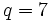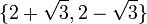# Element structure of special linear group:SL(2,7)

## Contents

View element structure of particular groups | View other specific information about special linear group:SL(2,7)

This article gives detailed information about the element structure of special linear group:SL(2,7), which is a group of order 336.

## Conjugacy class structure

In the table below, we consider the group as$SL(2,q), q = 7$. The information is stated for generic odd$q$ and then computed numerically for$q = 7$.

Nature of conjugacy class Eigenvalue pairs of all conjugacy classes Characteristic polynomials of all conjugacy classes Minimal polynomials of all conjugacy classes Size of conjugacy class (generic odd$q$) Size of conjugacy class ($q = 7$) Number of such conjugacy classes (generic odd$q$) Number of such conjugacy classes ($q = 7$) Total number of elements (generic odd$q$) Total number of elements ($q = 7$) Representative matrices (one per conjugacy class)
Scalar$\{ 1, 1 \}$ or$\{ -1,-1\}$$x^2 - 2x + 1$ or$x^2 + 2x + 1$$x - 1$ or$x + 1$ 1 1 2 2 2 2$\begin{pmatrix} 1 & 0 \\ 0 & 1 \\\end{pmatrix}$ and$\begin{pmatrix} -1 & 0 \\ 0 & -1\\\end{pmatrix}$
Not diagonal, Jordan block of size two$\{ 1, 1 \}$ or$\{ -1,-1\}$$x^2 - 2x + 1$ or$x^2 + 2x + 1$$x^2 - 2x + 1$ or$x^2 + 2x + 1$$(q^2 - 1)/2$ 24 4 4$2(q^2 - 1)$ 96 [SHOW MORE]
Diagonalizable over$\mathbb{F}_{q^2}$, i.e., field:F49, not over$\mathbb{F}_q$, i.e., field:F7. Must necessarily have no repeated eigenvalues. For$q = 7$:$\{ \sqrt{-1}, -\sqrt{-1} \}$,$\{ 2 + \sqrt{3}, 2 - \sqrt{3} \}$,$\{ -2 + \sqrt{3}, -2 - \sqrt{3} \}$ For$q = 7$:$x^2 + 1$,$x^2 - 4x + 1$,$x^2 - 3x + 1$ For$q = 7$:$x^2 + 1$,$x^2 - 4x + 1$,$x^2 - 3x + 1$$q(q - 1)$ 42$(q - 1)/2$ 3$q(q - 1)^2/2$ 126 PLACEHOLDER FOR INFORMATION TO BE FILLED IN: [SHOW MORE]
Diagonalizable over$\mathbb{F}_q$, i.e., field:F7 with distinct diagonal entries For$q = 7$:$\{ 2,4 \}$,$\{ 3,5 \}$ For$q = 7$:$x^2 - x + 1$,$x^2 + x + 1$ For$q = 7$:$x^2 - x + 1$,$x^2 + x + 1$$q(q+1)$ 56$(q - 3)/2$ 2$q(q+1)(q-3)/2$ 112 [SHOW MORE]
Total NA NA NA NA NA$q + 4$ 11$q^3 - q$ 336 NA